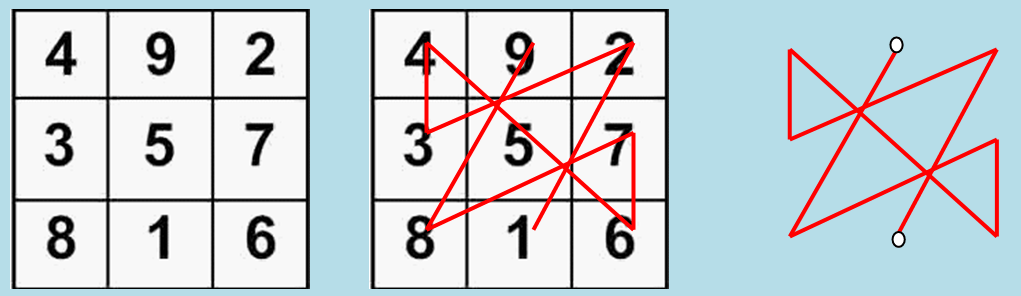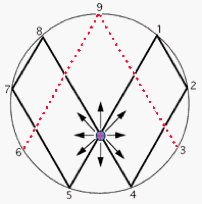# About the Sum of Things

The Lo Shu is a Chinese Model for Time and was used to design Cities, Temples, Cycles and Calendars.

The Lo Shu is a 3×3 Magic Square created by Fu Xi, the Founder of Chinese Civilization. Fu Xi lived around the time of the Great Flood.

The Magic Square was carried by a Turtle. The Turtle is the symbol of the Constellation Orion. Many Ancient Cultures claim that our Ancestors came from this constellation. The Square of Lo Shu is also referred to as the Magic Square of Saturn or Chronos (Time).

According to Chinese Creation Myth  the world started with 無極 (wuji: nothingness), the Tao. Out of the Tao, the Egg of the Zero (0) and the Supreme Pure One (1) emerged. In the next step the Trinity (3), the Three Pure Ones, was created.

The Tao produced One; One produced Two; Two produced Three; Three produced All things (Lao Tsu, Tao Te Ching, 42).

The Trinity is represented by a Triangle. A combination of Two Triangels is a Square. The combination of Two (2) Triangels is also the Symbol of the Heart Chakra, the merge of Heaven, the Upper Triangel and Earth, the Lower Triangel.

The most complex Yantra (“machine”), the Sri Yantra, contains 43 Triangels. The Sri Yantra is determinated by the Bronze Mean. The Bronze Mean is a generalization of the Sequence of Fibonacci based on the Trinity. The Bronze Mean generates Quasi-Crystals and Penrose Tilings.

The Lo Shu Magic Square not only shows the Connection between the Four Forces that came out of the Two Forces, Expansion and Compression, it also shows the connection between Heaven and Earth.

In the Magic Square of Lu Shu the Even Numbers (2,4,6,8 = 2 x (1,2,3,4 = 2×2)) are Black(Yang, Male). The Odd Numbers (1,3,5,7,9 = 3×3) are White (Yin, Female). The Sum of all the Numbers is 15 (3×5).

The Even Numbers are situated at the Corners of the Square.

When the numbers of each row are multiplied (8x1x6; 3x5x7; 4x9x2) they together total to 225 ((3×5)x(3×5)) — as do those of the columns (8x3x4; 1x5x9; 6x7x2).

The Magic Square is dominated by two numbers (2**1, 2**2, 2**3)  and (3, 2×3, 3**2), It contains four Prime Numbers (1,3,5,7). It also contains the first Perfect Number 6 (1+2+3 =1x2x3). 6 is also a Harmonic Divisor Number and has a Harmonic Divisor 2. The Sixth Day in the week is the day of Saturn, the God of Time.

The sequence 1,2,4,8, 1, 3,6,9, 27 is called the Tetrad of the Pythagorians and was, according to Plato, used by the Demiurg, the Creator of our Universe, to create the Soul. The Soul is a mixture of the Same (1), the Different (2), and Existence (3). The Tetrad was used to define the Harmony of the Spheres.

When you connect the numbers of the Lo Shu a pattern appears that is called the Seal of Saturn or the Dance of Yu.The Seal of Saturn or the Dance of Yu

The Sum of Magic Squares is a Magic Square and the product of a Magic Square M with a number a is again a Magic Square aM. Both Rules can be used to construct a Magic Square out of simple Binary Squares called Magic Carpets. One of these Magic Carpets is the Identity Matrix with One on the Diagonal.

Every Magic Square is related by Rotation and Mirroring to Eight other Magic Squares. This means that the central Lo Shu Magic Square with the Five in the Middle is related to Eight other Magic Squares with the other Numbers in the Middle. One of them contains the Nine and is called the Well.The Lo Shu and the Well
The Sum of numbers of the Well is 18 (2×9) and the sum of all opposites across the 8 Spoke Wheel = 9 (9+0, 8+1, 7+2, 6+3, 5+4). 2×9 means that there are Two Complementary Magic Squares. One Magic Square is Rotating with the Clock and the other Against the Clock.The Well is an Expansion and Contraction Cycle based on Powers of 2.

The Nine is the most important Number in the Magic Square. The Nine comes back in many ancient mythologies like the Egyptian Pesedjet and the Greek Ennead.

When you add the separate numbers of a number (54 = 5+ 4 = 9) the result is the Remainder when you divide the number by 9.  This number is called the Modulo 9 number.

The Powers of 2 (= Expansion) Mod 9 creates a pattern (1,2,4,8,(16)7,(32)5,(64)1,(128)2,…). This pattern is reversed when we divide by 2 (=Compression) (1/2 (0,5 =5), 1/4 (0,25 = 7), 1/8 (0,125 =8), 1/16 (0,625 = 4), 2,1).

The Expansion and Compression Pattern shows that the same pattern repeats itself when the Magic Square is expanded or compressed Six (6) Times. The 7th Step is the Same as the First Step.

After 2**6=64 (8×8) divisons of the cell a Specialized Cell is created, the stem cell. The same happens in Music.  The Game of Chess (Chiu King in China) with its 8×8 = 64 = 2**6 Playing Board contains every possible situation of the Game of Life.

The Two-Pattern does not contain the numbers 3,6 and 9. The number 9 is repeated every time when the pattern is expanded and compressed which means that Nine is the same as the Identity, the One (1+9 = 1). 3 and 6 Oscillate (1x2x3=6 and 1+2+3 =6) and  therefore represent the Duality (3+6 = 9, = the One).

If we combine the Expansion and Compression patterns a Torus (a Rotating Circle, The Wheel of Time) appears. The Zero (the Void) is in the Center and Contains the Vortex. The Vortex represents another Cycle (Black/White Hole, 3,6,9, the Wheel of Order) in which every structure/pattern is destroyed to start All Over Again.

If we subtract the numbers in the Square by the number of the Center, 5, a highly Symmetric Pattern emerges. This pattern shows that there are Two Mirror Universes both containing four Forces.

When we transform this pattern from Modulus 9 to Modulus 3 (the Trinity) we see that the number 4 equals 1 and represents a new beginning of the Cycle.

The Magic Square with the Zero in the Middle is surrounded by two Sequences of 123(4) with different Signs. The Signs are arranged in a Cyclic Pattern (- -, + -, + +, – +) that contains the Four Permutations of – and +. The Sum of the ++ and the — is -3 and +3, the Trinity. The Sum of +- en -+ is the One.

The Cyclic Pattern of + and – represents the Prisoners Dilemma that is solved by the Strategy of Tit for Tat. This strategy is applied by the Trickster, the God of Paradox, a concept of God in which God creates games that create games.

When you use the basic Addition and Multiplcation Rules of the Magic Square many important numbers and patterns  appear that are related to Ancient CalendersSymbols and Cycles.

About the Sum of Things

The Lo Shu Magic Square appeared a few years ago when I wanted to know more about Acupuncture.  Acupuncture is related to the Sheng Cycle and the Sheng Cycle is related to the Lo Shu. A Magic Square is the perfect way to visualize the Constraints that control the Four Forces in our Universe.

Soon after this discovery I found out the Lo Shu is related to the Trinity and the Bronze Mean which is an abstraction of the Fibonacci Sequence. The Bronze Mean generates the Sri Yantra and so called Quasi Crystals. Quasi Crystals explain Acupuncture.

A few weeks ago I started a discussion with Kim Veltman. Kim Veltman is the director of the Maastricht McLuhan Institute. He is researching Ancient Architecture and wrote a very interesting document called “Alphabets, Elements and Cosmologies”.

Ancient Alphabets are related to Numbers and Tones so Ancient Texts also represent Numerical Patterns and Music. You want be surprised to read that the Lo Shu showed itself in every Text Kim Veltman has analysed.

His document started a new inquiry into the pattern of the Lo Shu. During this research I detected the book “the Sum of Things” by Paul Martyn-Smith. Paul Martin-Smith worked together with Lee Burton.

This blog contains just a litte bit of the tremendous amount of information Paul Martin-Smith & Lee Burton have found about the essence of the I Tjing which is contained in just one simple 3×3 Magic Square.

If you want to know more the only thing you can do is to buy this book and start reading.

LINKS

About the Book “the Sum of Things” (Free Download)

About the I Tjing

The Calculations  behind the Number of Things

The Relationship between the Lo Shu and the I Tjing

About the Lo Shu Torus

About Saturn, the Son of the Sun

About the Trickster

How to construct Magic Squares out of Binary Squares (Magic Carpets)

The Sistine Chapel (Rome) and the Seal of Saturn

About the Relationship between the Lo Shu and Music

About the History of the Lo Shu

About the Wheel of Eternal Order and the Wheel of Time

About the Relationship between the Lo Shu and Chinese Alchemy

About the Great Flood

About the Orion Myth

About Plato, Pythagoras and the Lo Shu

About the Nothingness

About the Mirror Universe

About the Prisoners Dilemma

About the Bronze Mean

About the Sheng Cycle

Everything You want to Know about Sacred Geometry

About the Influence of the Lo Shu on Recent Art

The Lo Shu Square with Imaginary Numbers

## 3 thoughts on “About the Sum of Things”

1. This is fascinating, Thank you so much for your research.

2. Quincy Robinson

“Sum of Things” details two 3×3 squares that merit this level of investigation; one is the Lo Writing described here; the other is the Ho Tu River map.

3. Klasse Page. Danke.